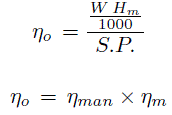Centrifugal Pump | Efficiency Of A Centrifugal Pump

EFFICIENCY OF A CENTRIFUGAL PUMP

Efficiency of a centrifugal pump is three types.

1) Manometric efficiency ( ηman )

2)Mechanical efficiency ( ηm )

3)Overall efficiency ( ηo )

In a centrifugal pump, Power is transmitted from the shaft of the electric motor to the shaft of the pump and then to the Impeller and from the Impeller the power is given to the water. So power is decreasing from the shaft of the pump to the ImpELler and then to the water. Read work done by a centrifugal pump on water. In this page, we will discuss the three types of efficiency of a centrifugal pump.

1)    Manometric efficiency ( ηman ):

The power at the Impeller of the pump is more then the power given to the water at outlet of the pump. The ratio of the power given to water at outlet of the pump to the power available at the impeller is known as manometric efficiency. Mathematically, manometric efficiency formula is written as: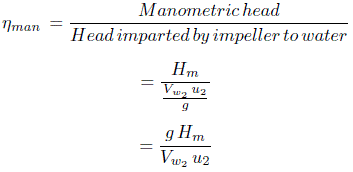The power at the Impeller of the pump is more then the power given to the water at outlet of the pump. The ratio of the power given to water at outlet of the pump to the power available at the impeller is known as manometric efficiency.

The power given to water at outlet of the pump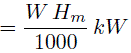The power at the impeller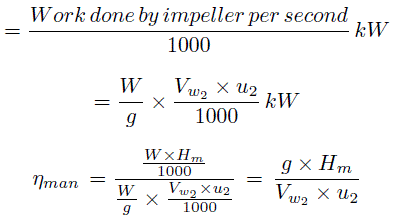2)    Mechanical efficiency ( ηm ):

Mechanical efficiency of a centrifugal pump is the ratio of the power available at the impeller to the power at the shaft of the centrifugal pump. The power at the shaft of the centrifugal pump is more than the power available at the impeller of the pump. Mathematically, mechanical efficiency formula is written as: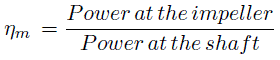The power at the impeller in kW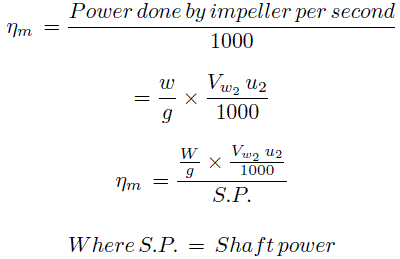3)    Overall efficiency ( ηo ):

Overall efficiency of a centrifugal pump is the ratio of the power output of the pump to the power input to the pump. The power output of the pump in kW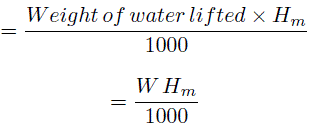Power input to the pump = Power supplied to the electric motor = S.P. of the pump